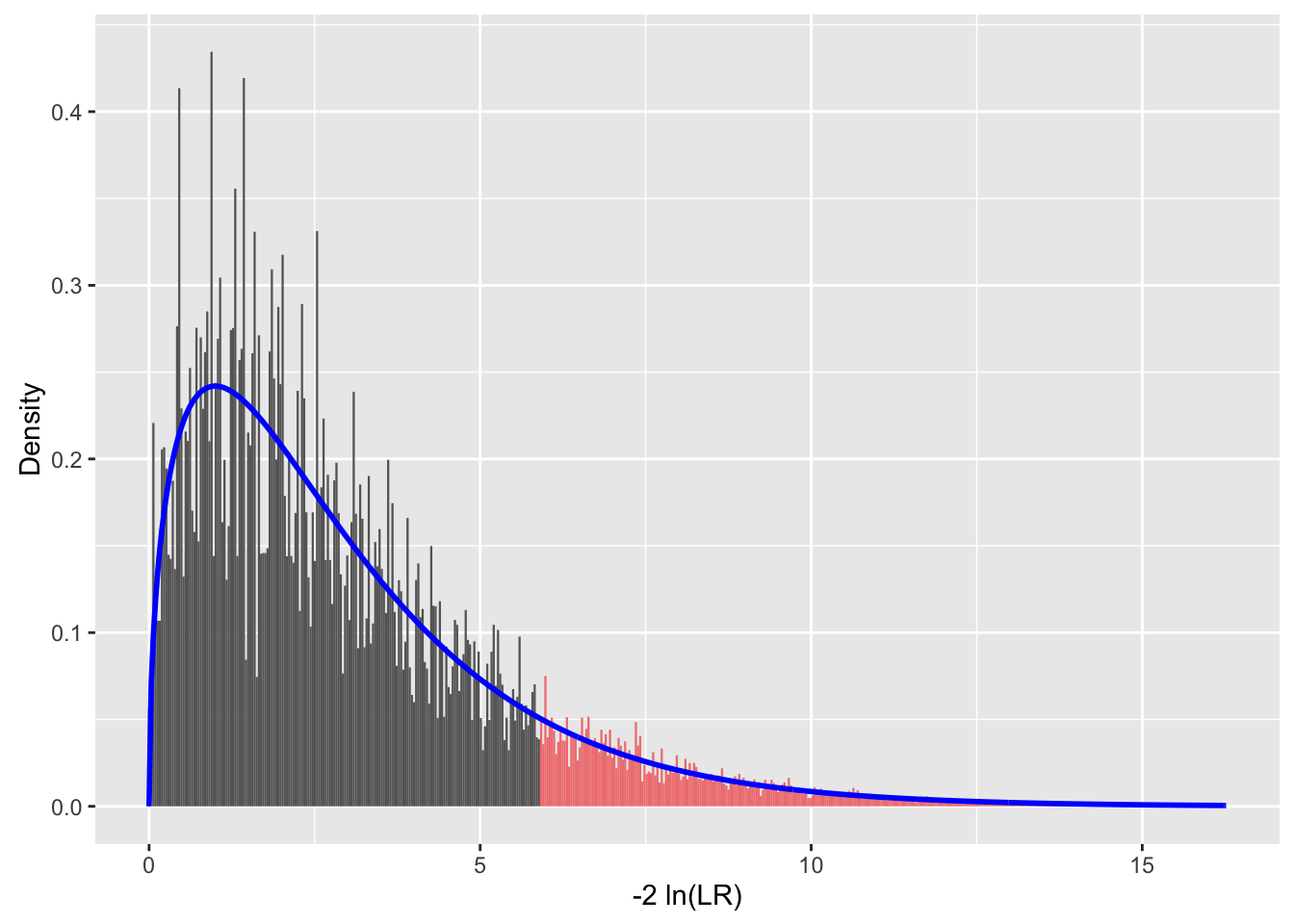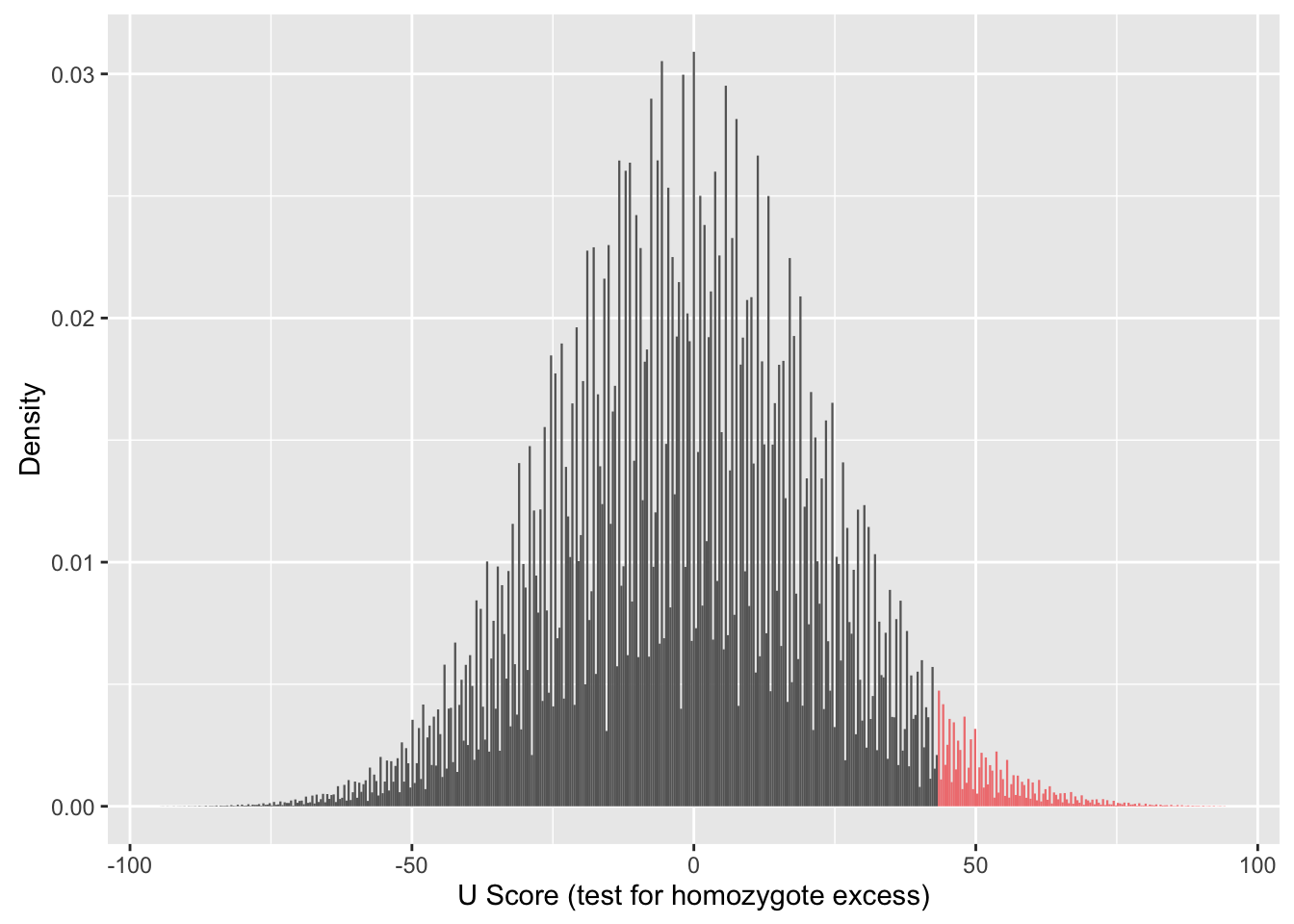# HWxtest – Exact Tests for Hardy-Weinberg Proportions With Multiple Alleles

### Main features

The HWxtest package tests whether a set of genotype counts fits Hardy-Weinberg proportions using the methods described by Engels (2009). The package’s main features are:

• Performs fast exact test with any number of alleles
• The $$P$$ value is determined using a choice of test statistics (see below). One option is to test specifically for an excess of homozygotes or heterozygotes.
• Can handle data sets with multiple loci or populations. It accepts data from other population genetics software: GenePop (Rousset, 2008), adegenet (Jombart, 2008), pegas (Paradis, 2010). Multithreading is an option when analyzing a list.
• Can perform either full enumeration test or Monte Carlo, depending on which is optimal for a given data set.
• Includes functions for determining the number of tables for a given set of allele counts

### Quick example

Suppose you sample 279 individuals from a population and determine their genotypes at a particular 3-allele locus. If the allele names are $$a_1, a_2$$ and $$a_3$$, the genotype counts might be: ${\bf{a}} = \left[ { \begin{array} {*{20}{c}} {{a_{11}}}&{}&{}\\ {{a_{21}}}&{{a_{22}}}&{}\\ {{a_{31}}}&{{a_{32}}}&{{a_{33}}} \end{array}} \right] = \left[ {\begin{array}{*{20}{c}} {83}&{}&{}\\ {49}&{18}&{}\\ {74}&{34}&{21} \end{array}} \right]\$

That is, we observed 83 homozygotes for allele $$a_1$$, 49 heterozygotes of genotype $$a_1/a_2$$, and so on. The genotype counts are in the lower half of a $$3 \times 3$$ matrix. (These values come from Morin, Archer, Pease, Hancock-Hanser, Robertson, Huebinger, Martien, Bickham, George, Postma, and Taylor (2012)) We’ll enter the numbers by rows in an R vector:

obs <- c(83, 49, 18, 74, 34, 21)

To test whether these numbers fit the Hardy-Weinberg proportions, call the function hwx.test

result <- hwx.test(obs)
result
##
## *****    Sample of 279 diploids with 3 alleles
## Full enumeration of 204350 tables to test for HW
##
## P value (LLR)   = 0.116908
## P value (Prob)  = 0.098767
## P value (U)     = 0.030499 (test for homozygote excess)
## P value (Chisq) = 0.109703

The output tells us that there are 204350 possible samples of 279 diploids with the same allele counts as the observed data. The $$P$$ values were computed by looking at each of those possibilities and adding up the total probability of all tables which deviate from HW by at least as much as the observed.

But why does it report four different $$P$$ values? The reason is that there are several ways one can measure just how deviant a particular outcome is compared to the HW expectation. These measures are explained in more detail below. Briefly, if you had no prior expectation that there might be too many homozygotes, then the first (LLR) $$P$$ value is recommended. However, if you had a prior suspicion that homozygotes would be in excess, such as from inbreeding, population admixture, null alleles, etc., then the third choice (U) would be preferable. The other two are included for comparison and for users who prefer them.

### Plot a histogram of the test statistic

The hwx.test function can also show a plot of how the test statistic is actually distributed, as opposed to its theoretical distribution for large samples. To do this, specify the number of bins to use in the histogram or just use T for the default of 500 bins:

hwx.test(obs, histobins=T)
##
## *****    Sample of 279 diploids with 3 alleles
## Full enumeration of 204350 tables to test for HW
##
## P value (LLR)   = 0.116908
## P value (Prob)  = 0.098767
## P value (U)     = 0.030499 (test for homozygote excess)
## P value (Chisq) = 0.109703The red area represents those outcomes found to be more extreme than the observed data by the LLR criterion. In other words, the red area represents the $$P$$ value. The blue curve shows the theoretical $$\chi^2$$ distribution for comparison. In this case the fit is pretty good.

We can do the same thing for the any of the other test statistics by specifying the statName:

hwx.test(obs, histobins=T, detail=0, statName="U")Note that setting the parameter detail to zero suppresses the re-printing of the numbers.

### Example with real data: bowhead whales

A set of data from P. Morin, Archer, Pease, Hancock-Hanser, Robertson, Huebinger, Martien, Bickham, George, Postma, and Taylor (2012) includes genotypes of 280 whales from one population and 49 from another. Each individual was classified at 51 genetic loci. Some of these loci had more than 20 alleles. We can test for Hardy-Weinberg fit for each locus and each population:

data(whales.df)
wtest <- hwx.test(whales.df)

Note that the format of the data is that of a data frame where each row has the ID of an individual whale followed by its population ID and the the genotype at each of the 51 loci. The result includes 102 individual tests, so let’s not print them all out. Instead, we’ll use the function hwdf to put the results in the form of a data frame, and look at just the first 10 in the list:

dfwhales <- hwdf(wtest)
dfwhales[1:10,]
##                           P-val(LLR)           ± SE     obs-LLR Method  Tables Trials   N k
## P1.BmC5R700_Y9101       6.276087e-01             NA  -0.9044246  exact    6160     NA 280 3
## P1.BmCATR205_R212       4.807641e-01             NA  -1.5072484  exact   15200     NA 280 3
## P1.BmCHYY286_R417       3.694901e-01             NA  -2.9062742  exact 1292854     NA 278 4
## P1.BmMPOR184_R284       1.169080e-01             NA  -2.9735065  exact  204350     NA 279 3
## P1.Bmys28Y154_R162      7.815520e-01             NA  -1.3732710  exact  176208     NA 277 4
## P1.Bmys387R245_R361     7.593449e-01             NA  -0.6840795  exact   11410     NA 279 3
## P1.Bmys404Y286_K316     3.593070e-02             NA  -4.3185064  exact  212551     NA 272 3
## P1.Bmys412R79_R463      2.346504e-06             NA -14.0551607  exact   12901     NA 280 3
## P1.Bmys42aK46_R225_K232 2.000000e-05 ± 1.414199e-05 -30.0615965  monte      NA  1e+05 263 7
## P1.Bmys43Y237_Y377      8.540000e-03 ± 2.909823e-04  -8.6795512  monte      NA  1e+05 277 4

The first column shows the population, such as “P1,” and locus, such as “BmC5R700_Y9101” of each sample. The second column is the $$P$$ value from the LLR criterion by default. N is the number of diploid individuals in the sample and k is the number of alleles.

Note that there is a standard error associated with the last two tests, but not the others. This is because those two tests were done by the Monte Carlo method rather than full enumeration. The hwx.test function decides which method to use based on how many tables would need to be examined in order to do the full enumeration. In these two samples, the number of tables would be 3.821481410^{22} and 152168317 respectively, and that would take too long. Instead, hwx.test performed 100,000 random trials to estimate the $$P$$ value in each case.

If you need a more precise $$P$$ value than the one provided by 100,000 trials of the Monte Carlo test, there are two ways to do it. One way is to simply increase the Monte Carlo sample size. Suppose we want to do that for the 7-allele case, P1.Bmys42aK46_R225_K232, but not for the whole data set. We can start by pulling out the genotype counts for that specific test. These genotype counts are contained in the wtest object and can be picked out as follows:

counts1 <- wtest$P1$Bmys42aK46_R225_K232$genotypes counts1 ## 001 002 003 004 005 006 007 ## 001 1 NA NA NA NA NA NA ## 002 1 19 NA NA NA NA NA ## 003 1 46 18 NA NA NA NA ## 004 1 24 33 3 NA NA NA ## 005 1 7 17 10 5 NA NA ## 006 0 14 19 7 14 4 NA ## 007 0 0 6 0 8 1 3 Now we can call hwx.test again, but increase the sample size to one million by changing the parameter B: hwx.test(counts1, detail=1, B=1000000) ## P value (LLR) = 6e-06 ± 2.4495e-06 The standard error of the $$P$$ value is now considerably smaller. For the other sample, P1.Bmys43Y237_Y377, the number of tables is not as huge, and we can obtain an exact $$P$$ value by increasing the cutoff number. The following tells hwx.test to perform the full enumeration test instead of the Monte Carlo unless the number of tables exceeds 2 billion: counts2 <- wtest$P1$Bmys43Y237_Y377$genotypes
hwx.test(counts2, detail=1, cutoff=2e8)
## P value (LLR) = 0.008886

This time, the $$P$$ value is reported without a standard error, indicating that an exact test with full enumeration was performed.

The results contained in wtest includes $$P$$ values for all four criteria. So, for example, if we were interested in the U test rather than the LLR we could have specified that in the data frame call:

hwdf(wtest, statName="U")[1:10,]
##                             P-val(U)           ± SE       obs-U Method  Tables Trials   N k
## P1.BmC5R700_Y9101       3.043444e-01             NA -14.7089179  exact    6160     NA 280 3
## P1.BmCATR205_R212       1.131530e-01             NA -29.1880342  exact   15200     NA 280 3
## P1.BmCHYY286_R417       3.130063e-01             NA   9.2963636  exact 1292854     NA 278 4
## P1.BmMPOR184_R284       3.049876e-02             NA  43.7794167  exact  204350     NA 279 3
## P1.Bmys28Y154_R162      3.123711e-01             NA -13.6939786  exact  176208     NA 277 4
## P1.Bmys387R245_R361     5.304596e-01             NA  -0.8355486  exact   11410     NA 279 3
## P1.Bmys404Y286_K316     2.630036e-02             NA -45.3982676  exact  212551     NA 272 3
## P1.Bmys412R79_R463      1.403760e-07             NA -90.6984792  exact   12901     NA 280 3
## P1.Bmys42aK46_R225_K232 4.040000e-03 ± 0.0002005911 128.7425357  monte      NA  1e+05 263 7
## P1.Bmys43Y237_Y377      3.198100e-01 ± 0.0014748951  10.5665228  monte      NA  1e+05 277 4

When using the U test, it is important to pay attention to the sign of U. If it is negative, as in the first two samples, the test is specifically for heterozygote excess. If it is positive, then we are testing for homozygote excess. This point will be discussed further below.

Published data with genotype frequencies are often available in the GenePop format (Rousset, 2008). These data can be easily converted to genind objects and then fed to hwx.test. For example, Hart, Popovic, and Emlet (2012) provide a supplemental file containing genotype counts at two loci in two populations of sea urchins. We can pull those numbers in directly from the online source and analyze them as follows:

urchin.url <- "http://tinyurl.com/ku4fq7m"
urchin.data <- genepop.to.genind(urchin.url)
hwdf(hwx.test(urchin.data))

### Standard asymptotic tests are often inaccurate

Most introductory genetics classes teach that the way to test for Hardy-Weinberg proportions is the standard $$\chi^2$$ test with $$k(k-1)/2$$ degrees of freedom. (I am guilty of this myself in teaching Genetics 466 at the University of Wisconsin!) The problem is that with real data and multiple alleles, there are often rare alleles which make the asymptotic test unreliable. For example, let’s compare the actual $$P$$ values for the bowhead whale data as computed in the previous example with the corresponding $$P$$ values from the ordinary $$\chi^2$$ test. First we make a data frame from wtest and extract the true $$P$$ values from the first column and the approximations from the $$10^{th}$$ column:

df <- hwdf(wtest, showAsymptoticX2=T)
pLLR <- df[]
pAsy <- df[]
par(mfcol=c(1,2))
plot(pLLR, pAsy, xlab="True P value", ylab = "Asymototic approximation")
abline(0,1)
lim <- c(0,.2)
plot(pLLR, pAsy, xlim=lim, ylim=lim, xlab="True P value", ylab="")
abline(0,1)